search: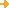advanced search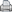printer-friendly version

Title: Famous Equations and Inequalities
Url: http://www.math.utah.edu/~alfeld/math/equations/equations.html
Creator: Alfeld, Peter
Publisher: University of Utah
 Description: An idiosyncratic and personal selection of particularly important or particularly intriguing mathematical equations, not all of them complicated. Mathematical Constants; The definition of Pi; The definition of e; A differential equation; The Pythagorean Theorem; The Fundamental Theorem of Calculus; Taylor Series; Eigenvalue Problems; Linear Systems; A Variational Principle; The Mandelbrot Set; Loan Payments; The Triangle Inequality; Reverse Triangle Inequality; Cantor's Theorem. Also equations for physics: Energy, mass, and the speed of light; Gravity.
LC Classification: Science -- Mathematics -- Algebra -- Number theory
Science -- Mathematics -- Algebra -- Theory of equations
Science -- Mathematics -- Analysis -- Differential equations
Science -- Mathematics -- Analysis -- Differential equations -- Differential-algebraic equations
Science -- Physics -- Mathematical physics -- Special topics, A-Z -- Difference equations
GEM Subject: Mathematics
Mathematics -- Calculus
Mathematics -- Geometry
Mathematics -- Algebra
Mathematics -- Functions
Mathematics -- Number sense
Key Concept: Mathematics -- Differential equations
Physics -- Gravity and gravitation
Physics -- Mass
Physics -- Speed
Date Issued: 1998
Format: Document -- HTML
Audience: Educator
Education Level: High School
Middle School
Language: English
Access Rights: Free access
 ScreenshotFull Image
Source: Math Forum
Full Record Views: 74
Resource URL Clicks: 7
Cumulative Rating: NOT YET RATED
 Report a Problem with this Resource Record

(no comments available yet for this resource)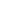forget your password?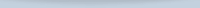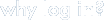Save, organize, and share resources that you find.

Subscribe to bulletins

It's easy, fast, and FREE!get your own loginAMSER =
FREE ONLINE
RESOURCES
for the
CLASSROOM